# Formulas for Set Theory

## Set theory and its Formula

Set theory is a branch of mathematical logic that studies sets, which informally are collections of objects. Although any type of object can be collected into a set, set theory is applied most often to objects that are relevant to mathematics. Formulas for Set Theory are very useful in terms of exams.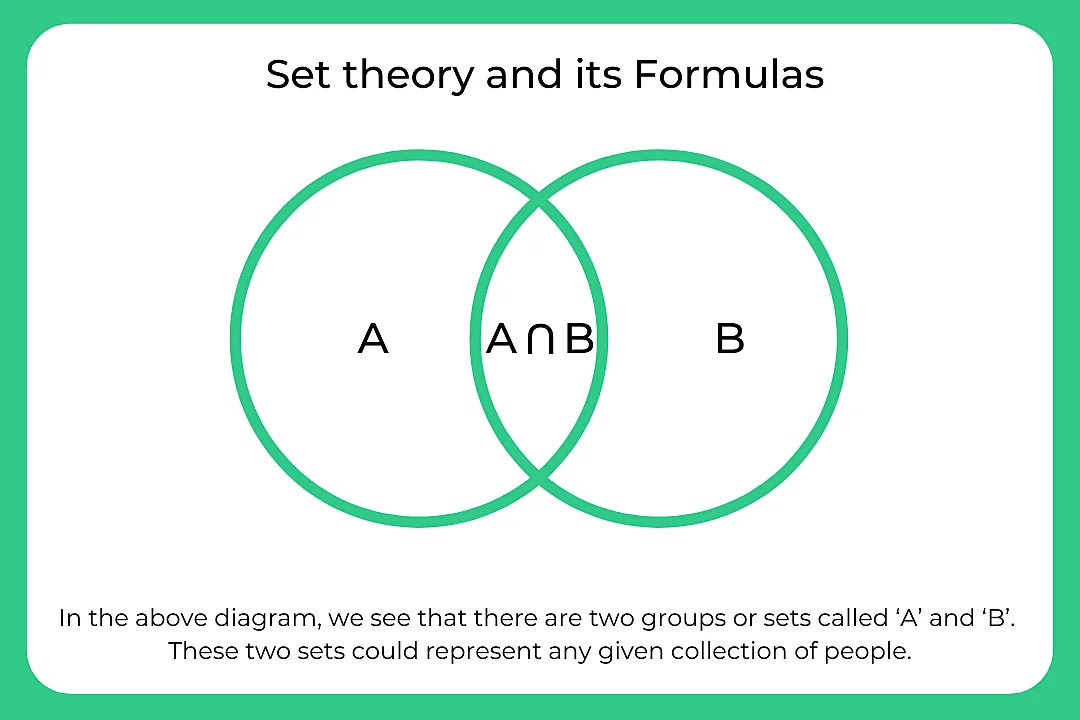### Formulas for Set Theory

Notations used in set theory:

• n(A) – Cardinal number of set A.
• n(A) – Cardinality of set A.
• $\overline{A}$ = Ac – complement of set A.
• U – Universal
• A ⊂ B – Set A is proper subset of subset of B.
• A ⊆ B – Set A is subset of set B.
• ∅ – Null set.
• a ∈ A – element “a” belongs to set A.
• A ∪ B – union of set A and set B.
• A ∩ B – intersection of set A and set B.

### Formulas

1. If A and B are overlapping set, n(A ∪ B) = n(A) + n(B) – n(A ∩ B).
2. If A and B are disjoint set, n(A ∪ B) = n(A) + n(B).
3. n(A) = n(A ∪ B) + n(A ∩ B) – n(B).
4. n(A ∩ B) = n(A) + n(B) – n(A ∪  B).
5. n(B) = n(A ∪ B) + n(A ∩ B) – n(A).
6. n(U) = n(A) + n(B) – n(A ∩ B) + n((A ∪ B)c).
7. n((A ∪ B)c) = n(U) + n(A ∩ B) – n(A) – n(B).
8. n(A ∪ B) = n(A – B) + n(B – A) + n(A ∩ B).
9. n(A – B) = n(A ∪  B) – n(B).
10. n(A – B) = n(A) – n(A ∩ B).
11. n(Ac) = n(U)- n(A)

### Related Banners

Get PrepInsta Prime & get Access to all 200+ courses offered by PrepInsta in One Subscription

## Some Examples based On Above Formulas:

Question 1: If A = { 1, 4, 5, 8} and B = { 6, 8, 10, 12}. Find A ∩ B.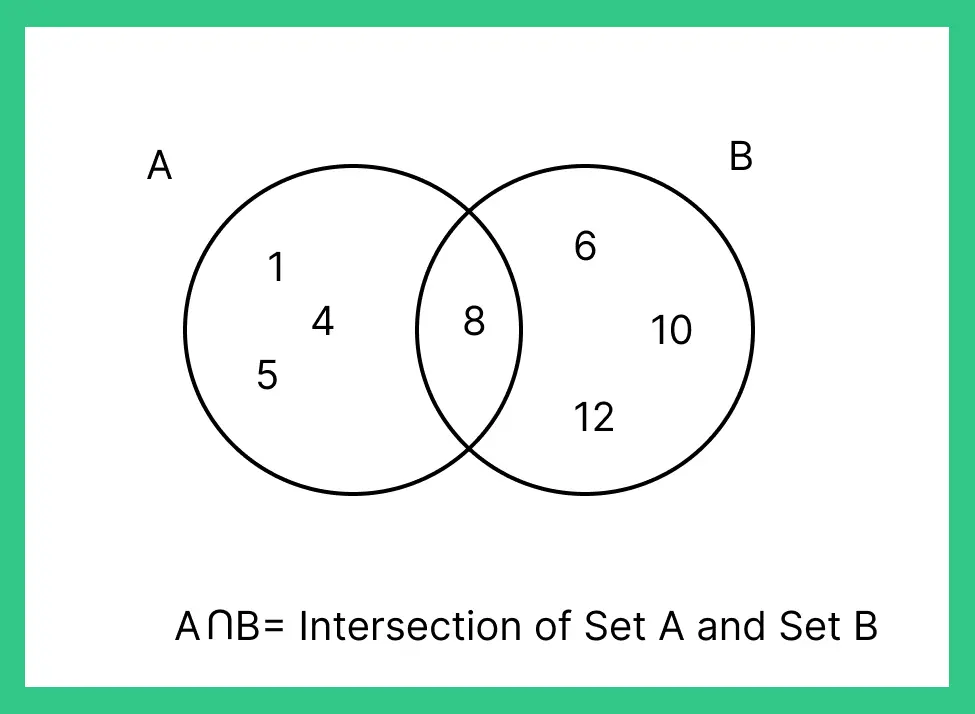Question 2: Check whether A={8,7,6,9} and B={9,6,7,8} are equal sets?

Answer: Yes they are equal sets since all the elements of A are present In B.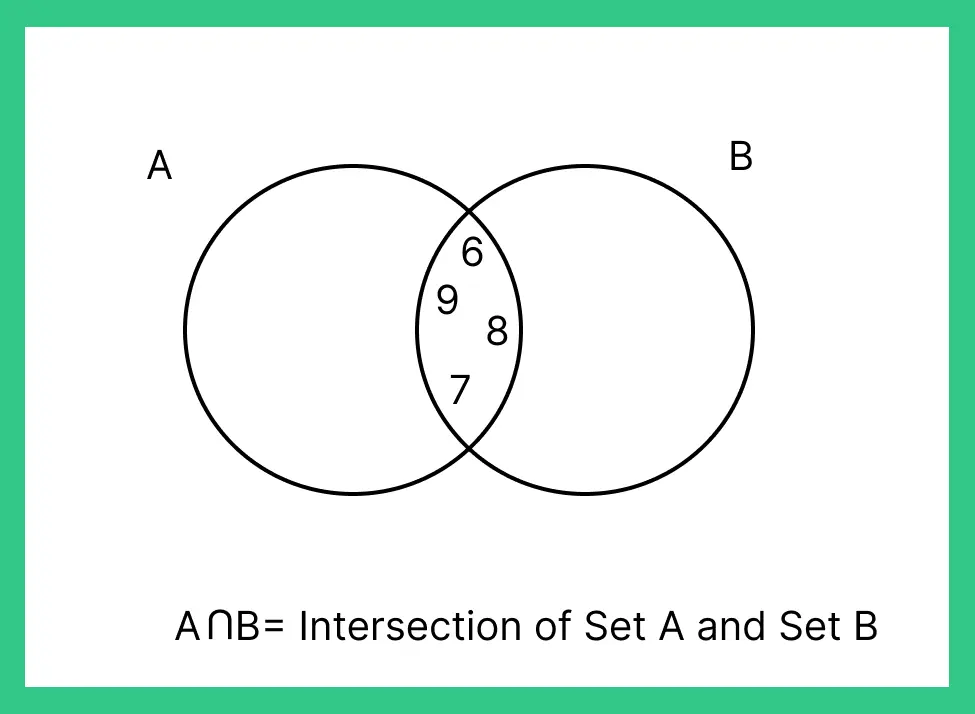Question 3: If A= { 7,8,0,6,8} B = { 5,6,7,8,9,0} Find A-B and B-A?

Answer: A-B= Elements in set a but not in b = { } null:

B-A= Elements in set b but not in a= {5, 9}.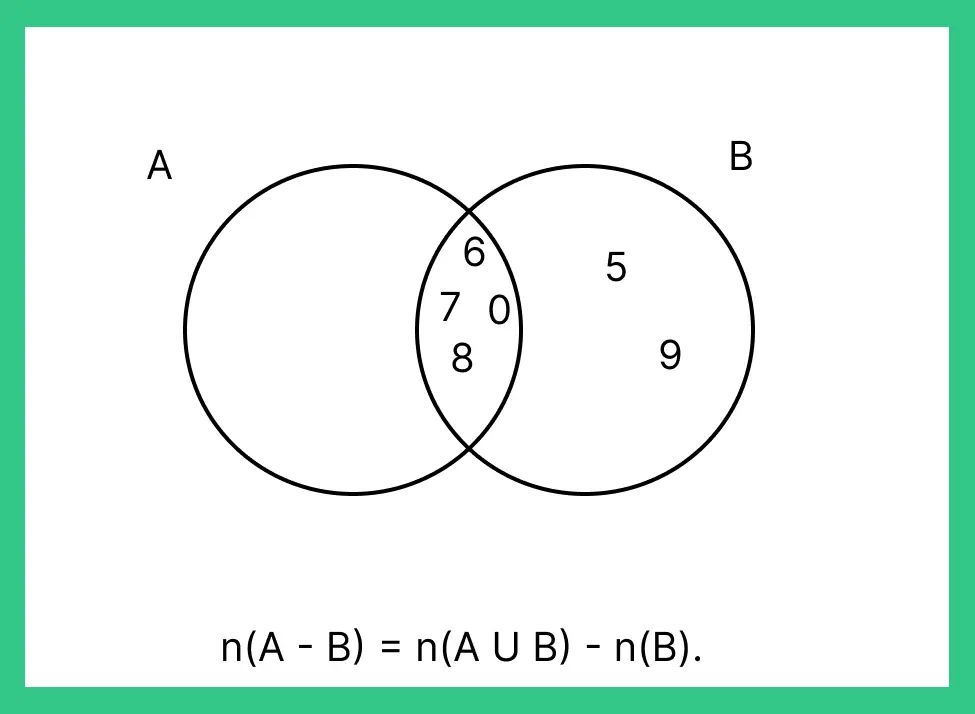Question 4:If A={1,2,3,4} B={3,5,6,9} and C={6,7,8,9} Find A(B ∪ C).

Answer: B ∪ C = {6,9}, A(B ∪ C) = { } null, as there is no element in common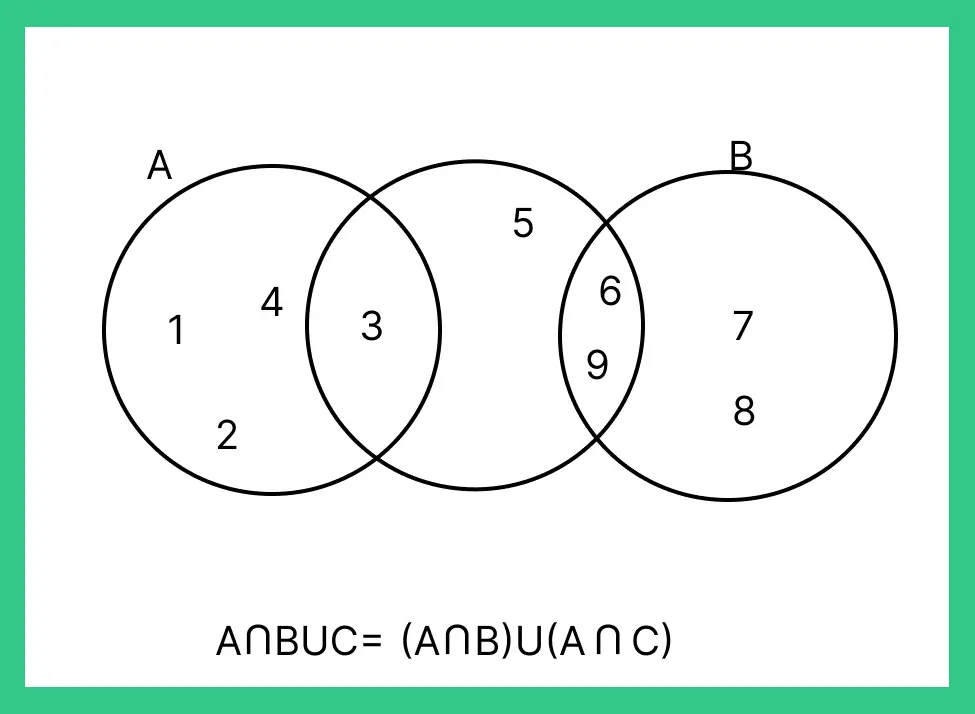Question 5: If U={ 1,2,3,4,5,6,7,8,9} A= {2,4,6} Find A’.

Answer: A’ means all the elements except that in A.   {1,3,5,7,8,9}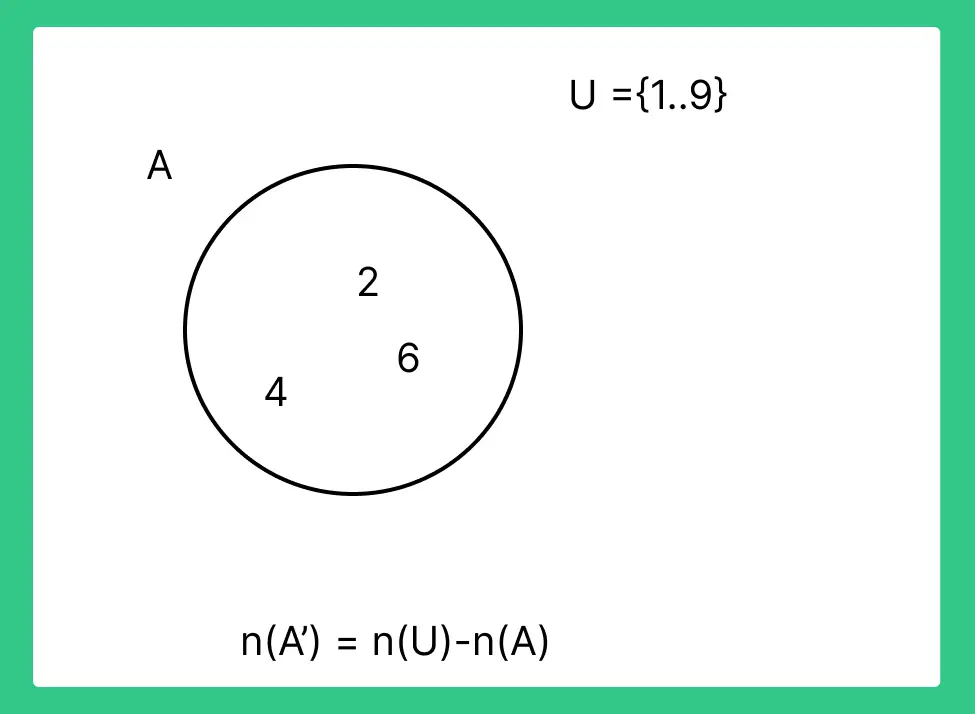## Get over 200+ course One Subscription

Courses like AI/ML, Cloud Computing, Ethical Hacking, C, C++, Java, Python, DSA (All Languages), Competitive Coding (All Languages), TCS, Infosys, Wipro, Amazon, DBMS, SQL and others